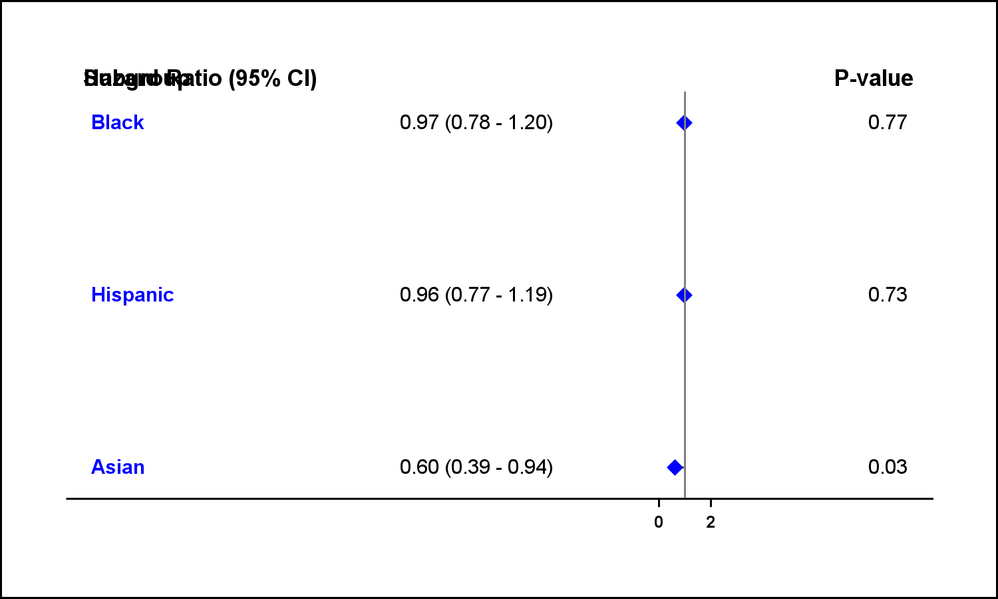## Modifying space between forestplot data

Hello everyone,

I have been trying to create a simple forest plot in SAS for my data. The ouptput looks good in my result viewer but its resolution is not great. I have tried severally to save it a picture format directly into my system but the space between the individual plots gets wider and the picture looks distorted. I have tried so many adjustments to the code such as changing the design height, the offsetmin and max but did not work.

I will appreciate your help. Also adding a label "higher mortality risk" and "lower mortality risk" did not work so I ran only the proc template to generate the result attached

Thank you

``````AS shown in my SAS result viewerWhen i save on my PC using ODS listing/************************************************************/
proc format;
value unicodeLabel
1 = "(*ESC*){unicode '2190'x} Lower mortality risk -"
2 = "- Higher mortality risk (*ESC*){unicode '2192'x}"
;
run;

data bottomLabel;
attrib textLeft format=unicodeLabel.
textRight format=unicodeLabel.;
labXLeft = 0.9; labXRight= 1.1; labY = 27; textLeft = 1; textRight = 2;
output;
run;

data forestfinal;
merge tcr.forest bottomLabel;
run;
/******************did not work******************************************/
proc template;
define statgraph forestAxisTable;
begingraph / designheight= 1.0in designwidth = 1.0in;

value '1' / textAttrs=(color=blue size=5 weight=bold);
value '2' / textAttrs=(color=blue size=5 weight=normal);
enddiscreteattrmap;

discreteattrvar attrvar=txtDAV var=id attrmap='text';

layout lattice / columns=1;

sidebar / align=top;
layout lattice / columns=3 rowweights=uniform columnweights = (0.3 0.42 0.28)
entry textAttrs=(size=8 weight=bold) hAlign=left "Subgroup"
hAlign=right "Hazard Ratio (95% CI)";
entry textAttrs=(size=8 weight=bold) " ";

entry textAttrs=(size=8 weight=bold) hAlign=right "P-value";
endLayout;
endsidebar;

/* Single cell with inner margins for left and right tables */
layout overlay / xAxisOpts=(display=(line ticks tickvalues)
tickValueAttrs=(size=6 weight=bold)
labelAttrs=(size=6 weight=bold)
linearOpts=(tickValuePriority=true
tickValueList=(0.0 0.5 1.0 1.5 2.0))
offsetMin=0.3 OFFSETMAX=0.5)
yAxisOpts=(reverse=true display=none) wallDisplay=none;

/* Left-side table */
innerMargin / align=left gutter=0.3in;
axisTable y=obsId value=SUBGROUP / textGroup=txtDAV
indentWeight=indentwt display=(values);
axisTable y=obsId value=GROUP / display=(values);
endInnerMargin;

/* Hazard Ratio plot */
referenceLine y=ref / lineAttrs=(pattern=solid thickness=15 color = white);
scatterPlot y=obsId x=mean / markerAttrs=(symbol=diamondFilled color = blue)
xErrorLower=LOW xErrorUpper=HIGH errorBarCapShape=none;
referenceLine x=1;

/* better indicator */
textPlot y=labY x=labXLeft text=textLeft / strip=true
position=left contributeOffsets=(yMin);
textPlot y=labY x=labXRight text=textRight / strip=true
position=right contributeOffsets=(yMin);

/* Right-side table */
innerMargin / align=right;
%let commonRightTableOpts = display=(values) showMissing=false
labelAttrs=(size=7 weight=bold);
/*axisTable y=obsId value=PCIGroup / &commonRightTableOpts;*/
axisTable y=obsId value=p_value  /  &commonRightTableOpts;
/**axisTable y=obsId value=p_value / &commonRightTableOpts
endInnerMargin;

endLayout; /* overlay */
endLayout; /* lattice */
endgraph;
end;
run;
ods listing image_dpi=200;
ods graphics / reset width=7.5in height=4.5in imagename='FIGURE4A';

proc sgrender data=tcr.forest2 template=forestAxisTable;
run;

``````

4 REPLIES 4

## Re: Modifying space between forestplot data

I have moved this topic to 'Graphics Programming' board.

This is indeed visual analytics ( or visual analysis strictly speaking )

, but it has nothing to do with the SAS product Visual Analytics.

Koen

## Re: Modifying space between forestplot data

Thank you very much for the clarification🙈 and for moving it to the right section

## Re: Modifying space between forestplot data

Are you running the code in SAS Studio, Enterprise Guide, DMS SAS, other?

Per the 'resolution', have you tried increasing where you're using image_dpi=200 ?

## Re: Modifying space between forestplot data

I'm running the program on SAS 9.4 windows. I tried modifying the resolution and size, but it was still the same.

Thank you

Discussion stats
• 4 replies
• 402 views
• 0 likes
• 3 in conversation